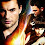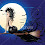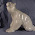## Tuesday, January 30, 2007

### Plot Algebra

Plot Algebraic Equations

Aphrodite's Curve Ball [H + H + A = LT]

To plot a love triangle, find the sum of the hero, heroine, and antagonist.

Boolean Secret Baby [H + H = (H + H)2 = H2 + 2H2 + H2 = H + 2H + H = H + H + H + H = B]

If the hero and heroine get together often enough, they will simplify their relationship, work out all their problems, and have a baby in last chapter.

Habeas Crime Fictionous [P / BLI + ES / TA = DB]

Protagonist divided by bimbo love interest plus ethnic sidekick divided by thuggish antagonist equals dead body.

Janus's Law of Duplicity [PT + RH = -A, P = A]

If the plot twists and red herrings do not result in an antagonist, then the protagonist is the antagonist.

Moufang Switcheroo [(PL)(IA) = P(LI)A

If the protagonist shares a love interest with the antagonist, then the love interest is out to screw one or both of them.

Properties of the Pissed-off [P < C, P + M (?GS + CC?) > C]

The protagonist is less than conflict, but the protagonist plus motivation is greater than conflict, especially if great sex and a cute chick are added into the equation.

Unending Order of the Infinite Series [P + . . . . + (C/(infinity)).FI = (number of open threads/2). (first + last motivations)=(infinity/2).(P+(C/(infinity)).FI)=(infinity/2).(P/(infinity)).A =(A-P)/Advance = KJA]

The bestselling fiction series shall be written until the publisher goes belly-up or the author drops dead, in which case Kevin J. Anderson will take over writing it.

(And they said that I'd never use it after high school. Ha.)

Extra credit: write an algebraic equation for your WIP or favorite novel in comments.

1.Anonymous7:20 AM

I always sucked at math.

the most complicated i could do would be

h+h+pt-bg= HEA

to get the happy ever after, add the hero, heroine and the plot twist, minus the sum of the bad guys.

;p

2.Anonymous7:57 AM

Okay, great, now my mind is trying to develop derivitives out of my current plots - and there's no way to type derivitives on a computer. (Math is thrown out, and my brain goes to Calculus - which I hated beyond all reason?!)

{[(P1+P2)/P2]-A}+SFC=TSFCE

The combined efforts of Protagonists 1 and 2, divided by the irritations of Protagonist 2, minus the actions of the Antagonist, plus the necessity of the scary fuzzy critter yields the typical science fiction cliff ending. ^-*

3.Anonymous8:18 AM

LOL this is fun. Let's see, mine would be: {Hi + He/A) - (PT + BA) = C/SP

Or, the heroine plus the (hero divided by the antagonist, as in, he is both) minus the plot twists plus the bigger antagonist equals conflict divided by subplots.

Or something like that. I'm still trying to figure out how one character fits multiple slots.
Jess

4.I don't have a little "integral" sign handy:

The integral from 0 to "the end" of S(x)dx = Bx^2 + Mx + L where B=blood, M=mythology and L=love.

5.Joely's equation definitely fits her just finished WIP.

Mine's more like: (H + S) / (D + P + G) X xLH = HWIP

That's Heroine plus slave, divided by Dragon plus Phoenix plus Goddess, multiplied by however many Little Helpers I find along the way gets you Horrible Work In Progress.

6.Let's see... For my WIP, it would be:

(P + A)(HTP - DB10) = J

(Protag plus Antag) times(Horrible TownsPeople minus Dead Bodies to the tenth power) equals Justice.

;o)

7.Anonymous10:01 AM

Okay, I was always better in geometry than algebra -- if I can't see a picture I can't see the point. So my question(besides what the heck is an integral sign and what does it do anyway?) is if you are writing spiritual romance does this mean your love triangles have to be right triangles? I'm assuming isosceles romantic triangles would ideally have the equal sides as hero and heroine, which one would label "h"? And, are all obtuse triangles relegated to erotica?
JulieB

8.Umm.

What language are we speaking?

9.Anonymous11:47 AM

LOL!

Geez, I sucked at algebra, but let's see...

where
h=heroine
B=bitch
A=antagonist
SC=scary cult
GGP=gorgeous gaelic priest
H=hero
IWADASD=it was a dark and stormy demon...

10.The Wandering Plotline:

AnH[(H+A)+(S-Px)] = W

Anti-hero meets heroine, who is tangled with another man she doesn't realize is the antagonist.
Add in scary elements minus a plot (Px) and that's equal to the characters wandering aimlessly through the novel.

Now somehow or another, I must solve for X. *_*

11.By the way, I failed Algebra 1 most heinously. So I wouldn't dare rely on my own formula. :P

12.Anonymous5:41 PM

(DH+K+HW+RS)-24=NWIP

Or... Spouse+Child+Housework+Required Sleep minus hours in a day=no work in progress.

~Avaron

13.Anonymous5:51 PM

[I + M + W = P]

however

[I + M + Wa = -P]

In other words, Ideas plus motivation plus words equals progress.

However, Ideas plus motivation plus day job equals no progress.

14.AAaaaaaaagh! Math! Hate it! I barely passed Algy 1 & 2. Ugh! That was over 2 decades ago. *still shudders* The only good equation I know is:

IC + JDM = PP

Eating ice cream while watching a Johnny Depp movie equals a happy pity party. *lol*

15.I think this would qualify more as story Caculus but here it is:

(TW + PW)W / DJ + PK = NL

Or Thinking about writing plus planning what to write times writing divided by dayjob and psychokitty equals no life. Oh well. Must go write now.
Ann

16.Erm...

((H + H) + (H + AH) / V x (PDT)) x Du = S

Heroine plus a Hero plus the Heroine plus an Anti-hero, all divided by the Villain multiplied by Place, Distance and Time multiplied by Danger to the unknown, equals.... A Sequel!!!

17.I know my tagline is "It's all about the numbers." But, really, I'm only able to do one word after another in the most rudimentary way. And precision isn't my strong point. Only last week I was saying, "A word here. A word there. Before you know it, there’s the whole darn novel."

This is hardly the stuff of algebraic equations. I'm clearly still in kindergarten where this writing stuff is considered. :) I'm lucky I can count in order. At least I can do it to 100k. And then I can subtract. Which should net me my finished product (or net, in this case):

w - f - r = N

Words minus fluff minus repetition equals Novel.

18.These are great! I had to come back just to see what everyone came up with. ^_^ Tempest, make room for me on the couch and save some ice cream!

19.Anonymous10:13 AM

That KJA one is too funny.
Thanks PBW for some morning chuckles.